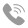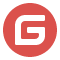# JavaScript 异步队列实现及拓展

### 异步队列

``a.then(() => b.then(() => c.then(...)))复制代码``

``````const queue = () => {
const list = []; // 队列
let index = 0;  // 游标

// next 方法
const next = () => {
if (index >= list.length - 1) return;

// 游标 + 1
const cur = list[++index];
cur(next);
}

// 添加任务
const add = (...fn) => {
list.push(...fn);
}

// 执行
const run = (...args) => {
const cur = list[index];
typeof cur === 'function' && cur(next);
}

// 返回一个对象
return {
run,
}
}

// 生成异步任务
const async = (x) => {
return (next) => {// 传入 next 函数
setTimeout(() => {
console.log(x);
next();  // 异步任务完成调用
}, 1000);
}
}

const q = queue();
const funs = '123456'.split('').map(x => async(x));
q.run();// 1, 2, 3, 4, 5, 6 隔一秒一个。复制代码``````

run 函数一旦执行，队列即开始 run。一开始执行队列里的第一个 async 函数，我们把 next 函数传给了它，然后由 async 函数决定什么时候执行 next，即开始执行下一个任务。我们没有并不知道异步任务什么时候才算完成，只能通过打成某种共识，来告知 queue 某个任务完成。就是传给任务的 next 函数。其实 async 返回的这个函数，有一个名字，叫 Thunk，后面我们会简单介绍。

### Thunk

thunk 其实是为了解决 “传名调用” 的。就是我传给函数 A 一个表达式作参数 x + 1，但是我不确定这个 x + 1 什么时候会用到，以及会不会用到，如果在传入就执行，这个求值是没有必要的。所以就出现了一个临时函数 Thunk，来保存这个表达式，传入函数 A 中，待需要时再调用。

``````const thunk = () => {
return x + 1;
};

const A = thunk => {
return thunk() * 2;
}复制代码``````

### 暂停

``````// queue 函数不变，
// async 加限制条件
const async = (x) => {
return (next) => {
setTimeout(() => {
if(x > 3) {
console.log(x);
q.run();  //重试
return;
}
console.log(x);
next();
}, 1000);
}
}

const q = queue();
const funs = '123456'.split('').map(x => async(x));
q.run();
//打印结果： 1, 2, 3, 4, 4，4， 4，4 一直是 4复制代码``````

``````const queue = () => {
const list = [];
let index = 0;
let isStop = false;

const next = () => {
// 加限制
if (index >= list.length - 1 || isStop) return;
const cur = list[++index];
cur(next);
}

const add = (...fn) => {
list.push(...fn);
}

const run = (...args) => {
const cur = list[index];
typeof cur === 'function' && cur(next);
}

const stop = () => {
isStop = true;
}

const retry = () => {
isStop = false;
run();
}

const goOn = () => {
isStop = false;
next();
}

return {
run,
stop,
retry,
goOn,
}
}

const async = (x) => {
return (next) => {
setTimeout(() => {
console.log(x);
next();
}, 1000);
}
}

const q = queue();
const funs = '123456'.split('').map(x => async(x));
q.run();

setTimeout(() => {
q.stop();
}, 3000)

setTimeout(() => {
q.goOn();
}, 5000)复制代码``````

### 并发

``````// 为了代码短一些，把 retry，goOn 先去掉了。

const queue = () => {
const list = [];
let index = 0;
let isStop = false;
let isParallel = false;

const next = () => {
if (index >= list.length - 1 || isStop || isParallel) return;
const cur = list[++index];
cur(next);
}

const add = (...fn) => {
list.push(...fn);
}

const run = (...args) => {
const cur = list[index];
typeof cur === 'function' && cur(next);
}

const parallelRun = () => {
isParallel = true;
for(const fn of list) {
fn(next);
}
}

const stop = () => {
isStop = true;
}

return {
run,
stop,
parallelRun,
}
}

const async = (x) => {
return (next) => {
setTimeout(() => {
console.log(x);
next();
}, 1000);
}
}

const q = queue();
const funs = '123456'.split('').map(x => async(x));
q.parallelRun();
// 一秒后全部输出 1, 2, 3, 4, 5, 6复制代码``````

## generator 与 co

### 简介 generator

``````// 一个简单的栗子，介绍它的用法

function* gen(x) {
const y = yield x + 1;
console.log(y, 'here'); // 12
return y;
}

const g = gen(1);
const value = g.next().value; // {value: 2, done: false}

console.log(value); // 2
console.log(g.next(value + 10)); // {value: 12, done: true}复制代码``````

gen 执行返回一个对象 g，而不是返回结果。g 跟其他 iterator 一样，通过调用 next 方法，保证游标 + 1，并且返回一个对象，包含了 value（yield 语句的结果），和 done（迭代器是否完成）。另外，yield 语句的值，比如上面代码中的 y，是下一次调用 next 传入的参数，也就是 value + 10，所以是 12.这样设计是有好处的，因为这样你就可以在 generator 内部，定义迭代算法的时候，拿到上次的结果（或者是处理后的结果）了。

### Thunk 实现

``````const coThunk = function(gen, ...params) {

const g = gen(...params);

const next = (...args) => { // args 用于接收参数
const ret = g.next(...args);   // args 传给 g.next，即赋值给上一个 yield 的值。
if(!ret.done) { // 去判断是否完成
ret.value(next);  // ret.value 就是下一个 thunk 函数
}
}

next(); // 先调用一波
}

// 返回 thunk 函数的 asyncFn
const asyncFn = (x) => {
return (next) => { // 接收 next
const data = x + 1;
setTimeout(() => {
next && next(data);
}, 1000)
}
}

const gen = function* (x) {
const a = yield asyncFn(x);
console.log(a);

const b = yield asyncFn(a);
console.log(b);

const c = yield asyncFn(b);
console.log(c);

const d = yield asyncFn(c);
console.log(d);

console.log('done');
}

coThunk(gen, 1);
// 2, 3, 4, 5, done复制代码``````

### promise 实现

``````// 定义 co
const coPromise = function(gen) {
// 为了执行后的结果可以继续 then
return new Promise((resolve, reject) => {
const g = gen();

const next = (data) => { // 用于传递，只是换个名字
const ret = g.next(data);
if(ret.done) { // done 后去执行 resolve，即co().then(resolve)
resolve(data); // 最好把最后一次的结果给它
return;
}
ret.value.then((data) => { // then 中的第一个参数就是 promise 对象中的 resolve，data 用于接受并传递。
next(data);  //调用下一次 next
})
}

next();
})
}

const asyncPromise = (x) => {
return new Promise((resolve) => {
setTimeout(() => {
resolve(x + 1);
}, 1000)
})
}

const genP = function* () {
const data1 = yield asyncPromise(1);
console.log(data1);

const data2 = yield asyncPromise(data1);
console.log(data2);

const data3 = yield asyncPromise(data2);
console.log(data3);
}

coPromise(genP).then((data) => {
setTimeout(() => {
console.log(data + 1); // 5
}, 1000)
});
// 一样的 2, 3, 4, 5复制代码``````

## async/await

``````const asyncPromise = (x) => {
return new Promise((resolve) => {
setTimeout(() => {
resolve(x + 1);
}, 1000)
})
}

async function fn () {
const data = await asyncPromise(1);
console.log(data);
}
fn();

// 那转化成 generator 可能就是这样了。 coPromise 就是上面的实现
function* gen() {
const data = yield asyncPromise(1);
console.log(data);
}

coPromise(gen);复制代码``````

asyncToGenerator 就是这样的原理，事实上 babel 也是这样转化的。

## 最后

CRMEB-暮白寒窗雪 最后编辑于2023-03-16 16:21:23

{{item.user_info.nickname}} {{item.user_info.is_threads ? '(管理员)' : item.user_id == thread_uid ? '(作者)' : ''}}

{{item.user_name}} {{item.user_info.is_threads ? '(管理员)' : item.user_id == thread_uid ? '(作者)' : ''}}

{{item.user_info.title}}
{{item.like_count}}

{{itemc.user_info.nickname}}

{{itemc.user_name}}

{{itemc.like_count}}

122
{{like_count}}
{{collect_count}}CRMEB-暮白寒窗雪 管理员596

837

15071内容精选{{item.title}}
{{item.created_at}}
{{item.fic_view_count}}

1、发布新帖子，每发布一条帖子可获得20个经验，每天最多可获取200个经验；

2、发布新回复，每发布一条回复可获得10个经验，每天最多可获取200个经验；

3、发布的帖子被管理员设置为精华帖，每被加精一次可获得10个经验，无上限；

4、发布帖子被回复一条，加2个经验，无上限；

5、发布帖子被点赞一个或发布评论被点赞加5个经验，无上限；

6、论坛首页大神积分排行榜，统计90天以内获取的累积经验值进行排序。

7、技术社区经验和官方商城用户经验同步，累计经验值会提升商城会员等级，购买官方商城产品享受对应会员等级折扣

{{item.label}} 首页头条 首页动态 加精 板块推荐 取 消 确 定咨询热线

400-8888-794开源下载帮助文档